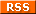Subscribe to Thomas mathematic adventures:# Sum of two squares and squares in arithmetic progression

The purpose of this applet is to find squares in Arithmetic Progression and this problem has been completely solved and can be done almost as fast as it takes to factorize the number. First you factorize the number. Then decompose it into a sum of two squares if possible and finally you can find the arithmetic progressions. There can be maximum 3 squares in an AP progression. Reading Squares in Arithmetic progression will explain the mathematics and how the program does the calculations.

 The applet will do the following: 1)Factorize the number n 2)Decompose n in sum of two squares in all possible ways (if possible) 3)Find all AP of squares which have n² as middle number.

 If you were using a Java-capable browser, you would see the applet instead of this paragraph.

# Limitations:

If the number has two or more very large prime factors (16+ digits) the factorization can take a long time. (using
Pollard's rho algorithm) If there are many (10000+) different arithmetic progressions the browser can run out of memory. You can configure the max memory for applets, but this is not an easy setting to change and is different in each browser. For numbers with less than 40 digits there should not be any problems. If the factorization has not been completed in 10 minutes there is a good chance it will take much longer or the applet has run out of memory. If factorization is your primary focus, go to the best factorization applet on the net: Factorization using the Elliptic Curve Method.

### Contact information:

If you have any questions or contributions to the problem you can contact me at thomas.egense@gmail.com